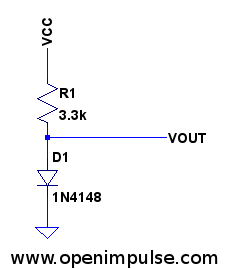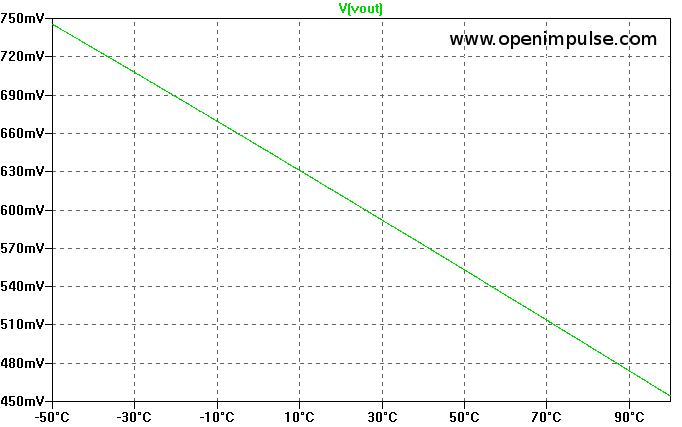# Low cost diode temperature sensorIf you need a temperature sensor but don’t have one at hand, here’s an easy way to create one, using a .. diode ! All what you have to do is to bias the diode with a current around 1 mA, and to measure it’s forward voltage drop.

The forward voltage of a silicon diode varies with about -2 mV / °C. So, basically the diode itself can be used as a temperature sensor. In order to have a good precision, you should perform a calibration by measuring the output voltage at a known temperature.

Then, you can compute the temperature as follows:

$$T = T_0 + (Vout_0 -Vout) \cdot 500$$

Where:
$$T_0$$ is the temperature at which you performed the calibration
$$Vout_0$$ is the output voltage measured at the calibration temperature

You can read the output voltage directly using the ADC from a microcontroller. Even the low cost microcontrollers have a 10-bit ADC, which would allow you to read the temperature with a precision of ± 1°C.

If you’re thinking about the performance of this sensor .. well it’s not bad at all. In the graph below you can see the dependence of the forward voltage with respect to the temperature:There are many commercial temperature sensors which are basically diodes, or transistors in diode connection.

## 2 thoughts on “Low cost diode temperature sensor”

1.Aman Rajbhandari on said:

$$T = T_0 + (Vout_0 -Vout) \cdot 500$$

What does \cdot 500\ mean?

•admin on said:

cdot is the multiplication sign, for some reason it does not display correctly there